## 双链表

#### 定义

typedef struct DNode
{
ElemType data;
struct DNode *prior, *next;


#### 初始化双链表和判断是否为空

bool InitDLinkList(DLinklist &L)
{
L = (DNode *)malloc(sizeof(DNode));
if (L == NULL)
return false;
L->prior = NULL; //带头节点的情况，头节点的prior永远指向 NULL
L->next = NULL;
return true;
}


bool Empty(DLinklist L)
{
if (L->next == NULL)
return true;
return false;
}


#### 向后插入元素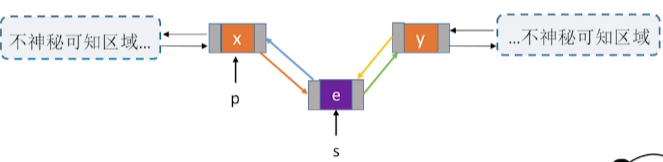1. s的后驱指针p的后驱，
2. p->next-prior 指向 s。
3. 将 p节点的后驱指针指向 s ,
4. s的前驱指针指向 p

// 后插操作
bool InsertNextDNode(DNode *p, DNode *s)
{
if (p == NULL || s == NULL)
return false; //异常输入
s->next = p->next;
if (p->next != NULL)
p->next->prior = s;
s->prior = p;
p->next = s;
return true;
}


#### 前向插入元素

bool InsertPriorDNode(DNode *p, DNode *s)
{
if (p == NULL || s == NULL)
return false; //异常输入
return InsertNextDNode(p->prior, s);
}


#### 删除后继节点q1. 将 p的后驱指针指向 q->next;
2. 判断q是否为最后一个元素，也就是，q.next==NULL?
3. 如果不是 q->next->prior 指向 p
4. 释放 q
5. 删除成功
bool DeleteNextDNode(DNode *p)
{
if (p == NULL)
return false;
DNode *q = p->next;
if (q == NULL)
return false;
p->next = q->next;
if (q->next != NULL)
q->next->prior = p;
free(q);
return true;
}


#### 销毁双链表

1. 从L->next;头元素开始删除，直到 L->next=NULL;
2. 释放L
3. L指向NULL
4. 完成
bool DestoryList(DLinklist &L)
{
while (L->next != NULL)
DeleteNextDNode(L);
free(L);
L = NULL;
return false;
}


#### 遍历节点

DNode* p=L.next;
while(p!=NULL)
{
//要实现的操作
p=p.next;
}


DNode* p=<某一个节点>;
while(p->prior!=NULL)//不处理头节点
{
//要实现的操作
p=p.prior;
}


## 循环链表

### 循环单链表

typedef struct LNode
{
int data;
struct LNode *next;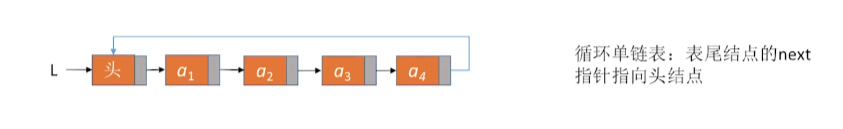#### 初始化和判断是否为空

// 初始化
{
L = (LNode *)malloc(sizeof(LNode));
if (L = NULL)
return false;
L->next = L; //头节点指向L
return true;
}
// 判断是否为空
{
if (L->next == L)
return true;
return false;
}


• 头指针指向 表尾
• 尾指针指向 头指针

### 循环双链表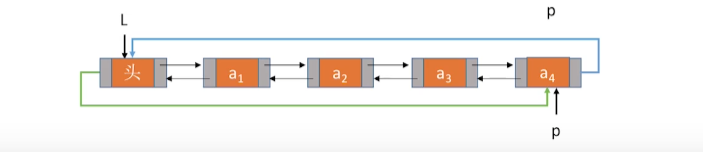typedef struct DNode
{
int data;
struct DNode *prior, *next;


#### 初始化和判空

// 初始化
{
L = (DNode *)malloc(sizeof(DNode));
L->next = L;
L->prior = L;
return true;
}
{
if (L->next == NULL)
return true;
return false;
}


#### 向后插入和删除

bool InsertNextDNode(DNode *p, DNode *s)
{
if (p == NULL || s == NULL)
return false; //异常输入
s->next = p->next;
p->next->prior = s;
s->prior = p;
p->next = s;
return true;
}


## 静态链表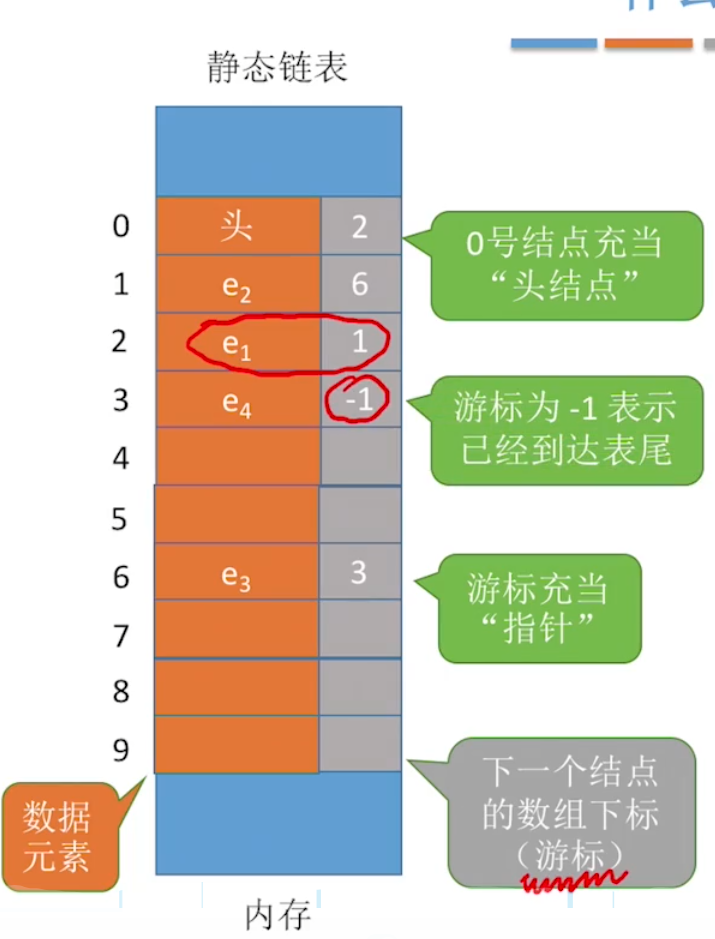#define MaxSize 10
typedef struct Node
{
int data;
int next;//下一个节点的游标


SLinkList a ;<=> struct Node{xxxx} a[MaxSize];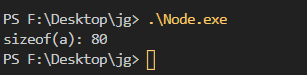#### 初始化

• 分配空间大小，
• 头指针指向分配的空间
• 头节点next指向NULL;

void InitSList(SLinkList &L)
{
for (int i = 0; i < MaxSize; i++)
L[i].next = MaxSize;
L.next = -1;
}


#### 向后插入e

1. 从头节点找到为序为 i-1第个结点,
2. 找到一个空的新节点，填入数据e,
3. 修改新的结点的next 为 i-i节点 的next
4. 修改i-1节点的 next为 e的next
5. 完成

#include <stdio.h>
#include <stdlib.h>
#define MaxSize 10
typedef struct Node
{
int data;
int next;
{
for (int i = 0; i < MaxSize; i++)
L[i].next = MaxSize;
L.next = -1;
}
bool InsertNext(SLinkList &L, int e, int i)
{
if (i < 1)
return false;

// 找到i-1个元素p
int p = 0;                   //当前遍历到的节点index
int j = 0;                   //当前遍历到第j个节点
while (p != -1 && j < i - 1) //p == -1 是表明表尾
{
p = L[j].next;
j++;
}
if (p == -1) //如果插入的位置大于当前长度
return false;
// 找到空缺位置，填入数据 e,带头指针情况
int n = 1; //插入元素的index
while (n < MaxSize)
{
if (L[n].next == MaxSize)
{
L[n].data = e;
break;
}
n++;
}
// 如果空间不够失败
if (n == MaxSize)
return false;
L[n].next = L[p].next;
L[p].next = n;
return true;
}


void printList(SLinkList L)
{
int p = L[p].next;
while (p != -1)
{
printf("L[%d].data:%d\n", p, L[p].data);
p = L[p].next;
}
}
int main()
{
InitSList(L);
InsertNext(L, 5, 1);
InsertNext(L, 6, 2);
InsertNext(L, 4, 1);
printList(L);
return 0;
}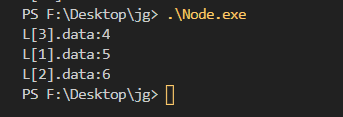#### 按元素查找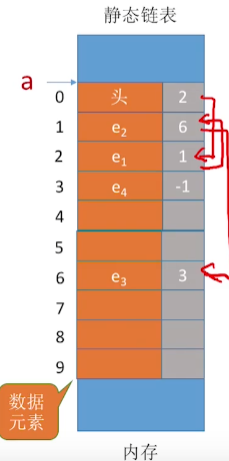p=L.next;
while(p!=-1)//不是表尾
{
//操作
}


#### 删除数据

1. 从头结点触发找到前驱结点（第i-1个）
2. 修改前驱结点的next
3. 删除结点的next值为MaxSize

1. 容量固定不变
2. 无法随机读取
3. 只能从头开始往后查

#### 适用场景

1. 不支持 指针的编程语言
2. 数据元素固定不变的场景 如 文件分配表 FAT，用来记录文件所在位置的表格